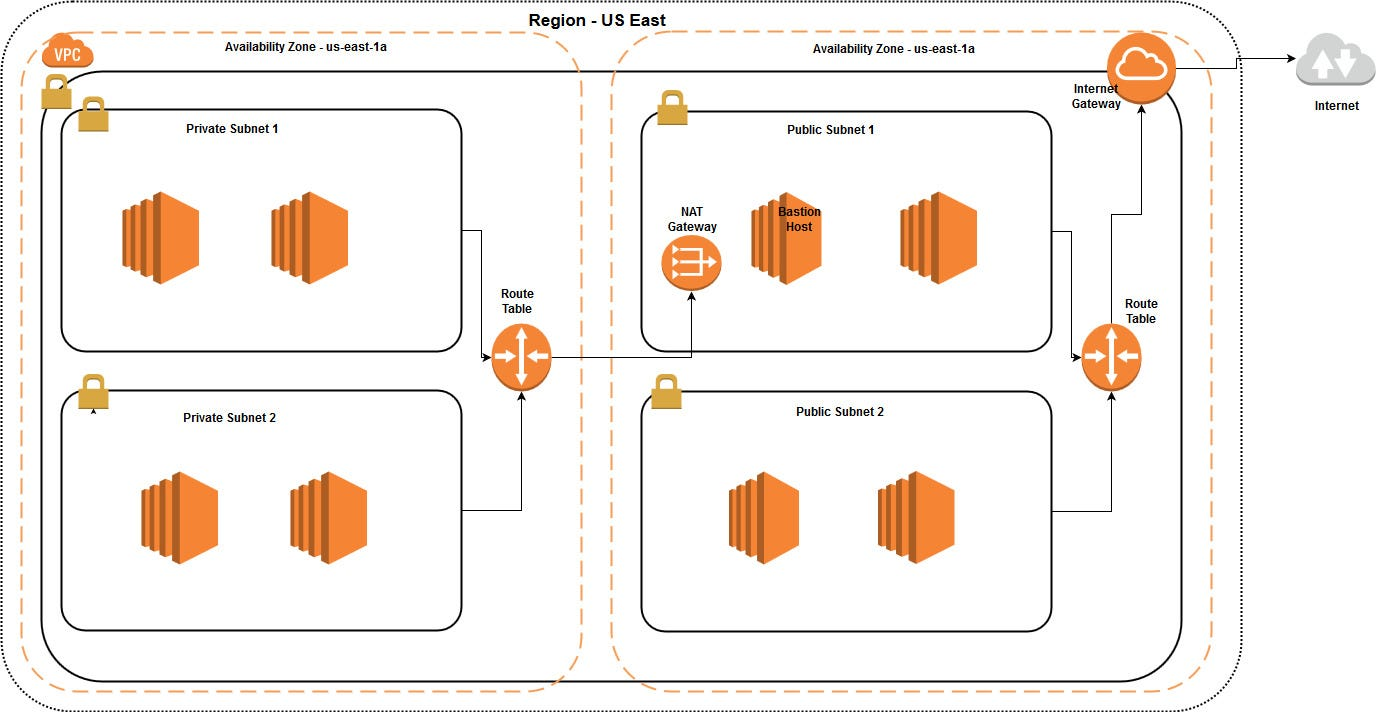# AWS VPC Subnets

VPC Design ArchitectureWe have to decide the design for the VPC base on the requirement.Here we are going to create VPC with two private subnets and two public subnets as mentioned in the above diagram. Let’s do the implementation step by step.
Step 01 : Design the VPC architecture
Decide the IP range for the VPC before creating the VPC. Here we are using 10.1.0.0/24 as the CIDR block.This CIDR block assigns 256 IP address for the VPC. Hence we are using 4 subnets for the VPC we have to divide the 256 IP addresses among 4 subnets. I’ll explain how to calculate number of IP addresses for the VPC and divide them between subnets.
CIDR block for the VPC -> 10.1.0.0/24
Number of IP address -> 2^(32–24) -> 2⁸ -> 256
Assuming equal number of IP addresses for the each subnet,
Number of IP addresses for a subnet = 256/4 = 64
Here is the IP address distribution for the above 4 subnets
Public Subnet 1 -> 10.1.0.0/26 (IP range — 10.1.0.0–10.1.0.63)
Public Subnet 2 -> 10.1.0.64/26 (IP range — 10.1.0.64–10.1.0.127)
Private Subnet 1-> 10.1.0.128/26 (IP range — 10.1.0.128–10.1.0.191)
Private Subnet 2 ->10.1.0.192/26 (IP range — 10.1.0.192–10.1.0.255)